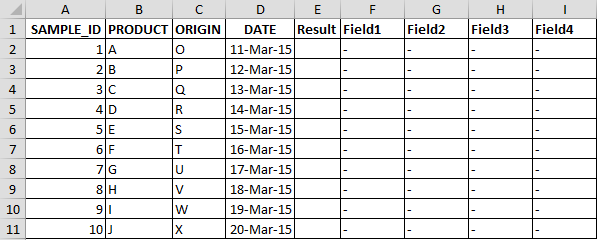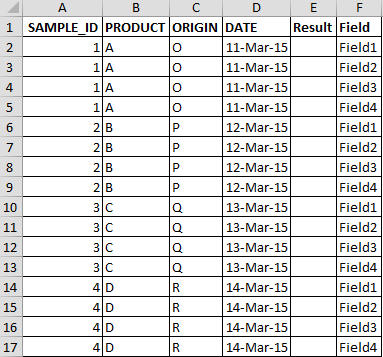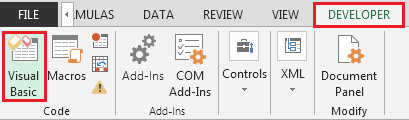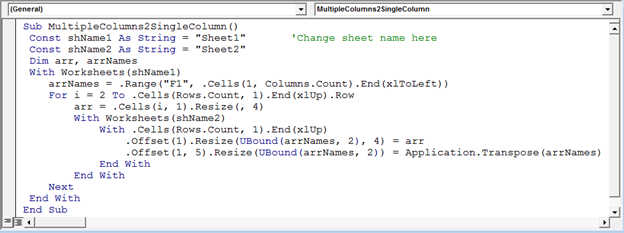# How to Combine Multiple Columns into Single Column Using VBA in Microsoft Excel

In case you have a requirement on combining multiple columns to on column & you did not have a clue then this whole article is for you. In this article we are going to learn how to combine multiple columns to one column using vba code.

From below snapshot:-Following is the snapshot of require output:-We need to follow the below steps:

• Click on Developer tab
• From Code group select Visual BasicEnter the following code in the standard module

Sub MultipleColumns2SingleColumn()

Const shName1 As String = "Sheet1"       'Change sheet name here

Const shName2 As String = "Sheet2"

Dim arr, arrNames

With Worksheets(shName1)

arrNames = .Range("F1", .Cells(1, Columns.Count).End(xlToLeft))

For i = 2 To .Cells(Rows.Count, 1).End(xlUp).Row

arr = .Cells(i, 1).Resize(, 4)

With Worksheets(shName2)

With .Cells(Rows.Count, 1).End(xlUp)

.Offset(1).Resize(UBound(arrNames, 2), 4) = arr

.Offset(1, 5).Resize(UBound(arrNames, 2)) = Application.Transpose(arrNames)

End With

End With

Next

End With

End SubAs you execute the macro; the macro will transfer the data from multiple columns to a single column.

In this way we can combine multiple columns data in a single column.1.